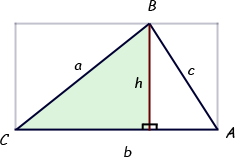SEARCH HOMEMath Central Quandaries & QueriesQuestion from Eileen, a student: Use a formal Statement/Reason Proof to prove the following.  Include a diagram, labeled appropriately. Given:  Acute triangle ABC, with a, b, c, being the respective opposite sides to angle A, angle B, angle C, and altitude, h, drawn from angle B to b. Prove:  The area of triangle ABC=1/2abSin C How would I do this??Hi Eileen.

There are many ways to do it. I would start by drawing the triangle, inside the rectangle (remember that the altitude h is perpendicular to the base b).First, you can say that the area of the rectangle is hb. Then you can reason that the triangle's area is ½hb since it neatly divides the rectangle into two pairs of congruent triangles.

Now look at the right triangle I've shaded. Can you find an expression for h in terms of a and C? If so, replace h in the ½hb calculation and you will have proved the solution.

Cheers,
Stephen La Rocque.Math Central is supported by the University of Regina and The Pacific Institute for the Mathematical Sciences.